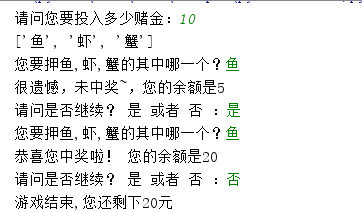# 用python编程 押宝游戏

# author : momo
'''

'''
import  random
a = int(input("请问您要投入多少赌金："))
list1 = ['鱼','虾','蟹']
print(list1)
while True:
if a>0:
b = input("您要押鱼,虾,蟹的其中哪一个？")
if b == random.choice(list1):
a = a+15
print("恭喜您中奖啦！ 您的余额是%d"%a)
else:
a = a - 5
print("很遗憾，未中奖~，您的余额是%d" % a)
if a>0:
c = input("请问是否继续？ 是 或者 否 ：")
if c=='是':
continue
elif c =='否':
break
else:
break
print("游戏结束,您还剩下%d元"%a)